Molecules of Life

Four major classes of biological molecules:

 Class of Molecule Composition Role in Human Body Lipids C, H, O (N, P) Membrane Structure, Fuel, Fuel Storage Carbohydrates (CHO's) C, H, O (N) Fuel, Fuel Storage, Cell-Cell Recognition Proteins C, H, O, N (S) Enzymes, Tissue Structure, Fuel Nucleic Acids (DNA, RNA) C, H, O, N, P Genetic Information Storage and Transfer

Lipids, Carbohydrates, Proteins, Nucleic Acids

Lipids:

1. Soluble only in hydrophobic (non-polar) solvents.

2. Ester bonds....

There are several subclasses of lipids:

1. Triacylglycerols (fats)

Triglycerols are formed via Ester Bonds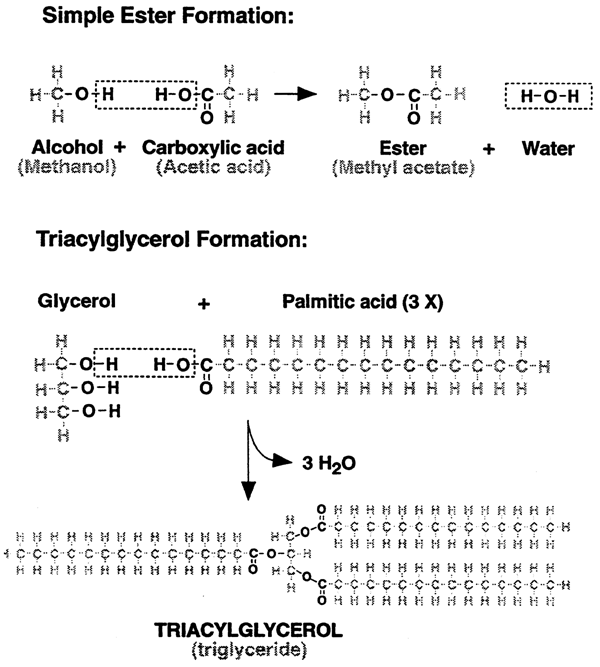2. Phospholipids

Have a tendency to form lipid bilayers (biological membranes).

Phosphoester and Phospholipid Formation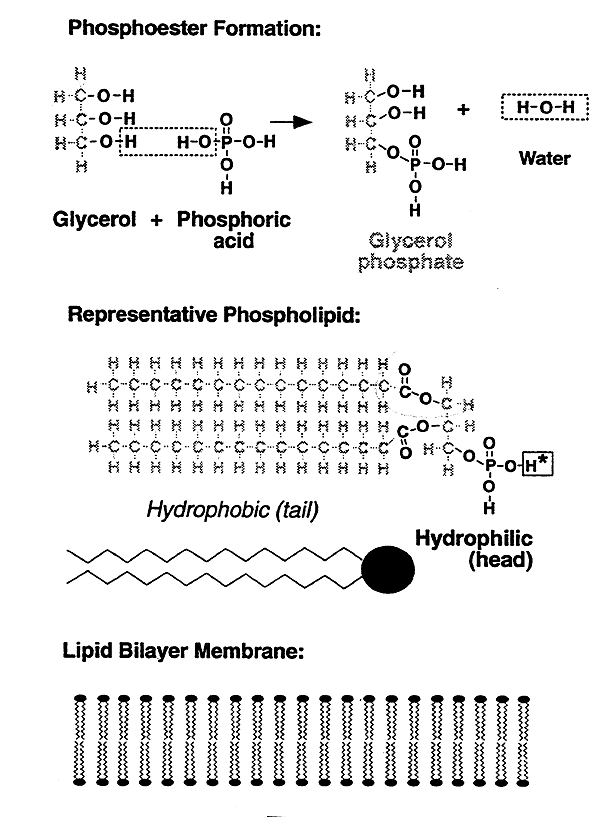Biological membranes (products of phospholipid formation). Like attracts like. "Hydrophobic" tails inside together, "hydrophilic" heads outside (H-bonding w/ water). Proteins: membrane spanning (channels), surface.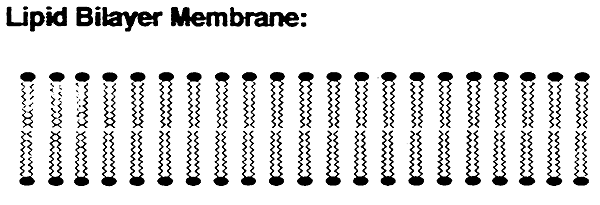3. Steroids- Cholesterol (rings, hydroxyl)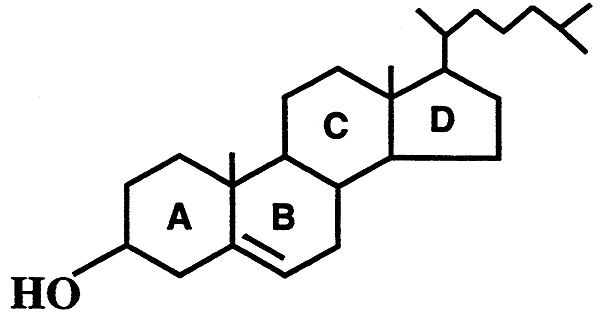Carbohydrates: or sugars

1. Water soluble

2. Glucose is the most abundant, occurs as a free sugar or as a polymer (glycogen) for storage

3. Structure of D-glucose (open chain, ring, H-bonding)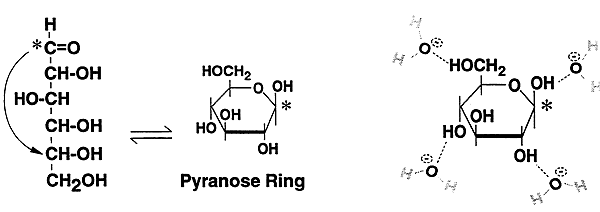Proteins:

1. Polymers of amino acids

2. Enzymes are proteins, catalysts of biological reactions

Nucleic Acids:

1. DNA / RNA

2. Information storage and transfer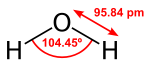Can water be considered an acid because of its ability to shed a proton:

H-O-H --> H+ + OH-

The frequecy with which this occurs in pure water is very low.....in fact only one water molecule in 500 million will be ionized at any time. Thus,

the molar concentrations of H+ and OH- are approx. 10-7 (divide the total molar concentration of water, which is ~55M by 500 million)

Special mathematical notation, by convention, the concentrations of H+ and OH- are expressed as:

-log [H+] = -log [10-7] = 7 = pH of pure water also,

[H+] [OH-] = 10-14 this allows us to calculate the [OH-] if [H+] is known

Acids:

Compounds capable of giving up a proton in solution. Strong acids such as HCl almost always exist in ionized form.

Most biological acids are significantly weaker than HCl. A good example is carboxylic acids. Since the difference in electronegativity is not as dramatic as in HCl, the tendancy of a carboxylic acid to give up its proton is much less.

Because of the great variety of craboxylic acids their tendancies to donate protons to solution also vary, so a mathematical decription like that for pH was developed called pKa.

The pKa is the pH at which the acid is half protonated and half deprotonated.

i.e.

CH3COOH(acetic acid) ----> CH3COO-(acetate ion) + H+, pKa = 4.8,

meaning that at pH = 4.8, the pool of acetic acid molecules exists half ionized.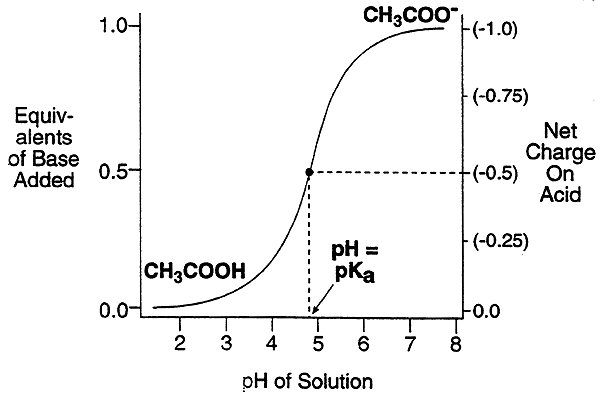In general, a way to describe all weak acids:

HA ---> A- + H+, pKa = ?? (dependent on electronegativity of A)

At pH values other than the pKa, the ratio of protonated to deprotonated or ionized form is something other than one. A convenient equation to calculate this ratio given the pH is the Henderson-Hasselbach equation:

pH = pKa + log [A-] / [HA]

This equation fits the definition of pKa ;

if pH = pKa, then log [A-] / [HA] = 0, and [A-] / [HA] = 1.

Other solutions to the Henderson-Hasselbach equation over a range of pH values can be displayed graphically.

-More than a pH unit below the pKa value, the acid molecules are mostly protonated. (charge = 0).

-More than a pH unit above the pKa, the acid molecules are mainly deprotonated. (charge = -1).

-Within a pH unit of the pKa value, the acid molecules are partially charged.

-Within a pH unit of the pKa value, the acid tends to resist a change in pH (the solution is buffered).